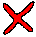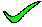The cardinality is the number of rows in the relation. If C is different from A by projection, then the number of columns changes, not the number of rows.

closeThe cardinality of the number of rows in the relation. Projection only effects arity.

closeThe arity is the number of columns in the relation. In projection, either the arity stays the same or is reduced. It can never increase using projection.

closeThe arity is the number of columns in the relation. In projection, either the arity stays the same or is reduced. The fact that C is different from A means this is the only true option.

closeThe cardinality is the number of rows in the relation. The arity is the number of columns in the relation. If C is different from A, then C must differ in the arity from A. In projection, either the arity stays the same or is reduced.

close

 A B C D E TELL ME NEXT INDEX

Relation C is a projection of relation A. Which of the following statements must be true in all cases where relation C is different from relation A?

1. The cardinality of C is greater than the cardinality of A
2. The cardinality of C is less than the cardinality of A
3. The arity of C is greater than the arity of A
4. The arity of C is less than the arity of A
5. None of the above